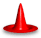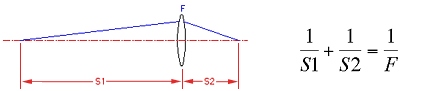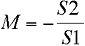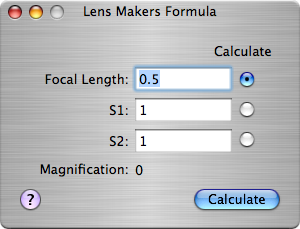OpticsCalc: Lens Maker's FormulaDescriptionThe lens makers formula relates the position of the object (S1) and image (S2) relative to a lens given the focal length of the lens.The calculator also computes the magnification of the image for this set of parameters. The magnification is computed as...The minus sign indicates that the image for a normal lens is inverted when compared to the object.

This page lets you enter any two of the three values and compute the third and the magnification. It is a manual computation page so you must fill in the known quantities, select the value you want to calculate by pressing the corresponding radio button and pressing Calculate.A Note On UnitsUnits are whatever the user wants, but they must be the same for all values.